When two or more forces act on a body, it is known as force system. If all these forces act on a single plane about any given point, it is known as coplanar forces.

A system of forces on a single plane either has same common line or they intersect at a common point. As per their line of action, the system of forces can be divided into two i.e., coplanar forces or non-coplanar forces as shown in Fig. 1.31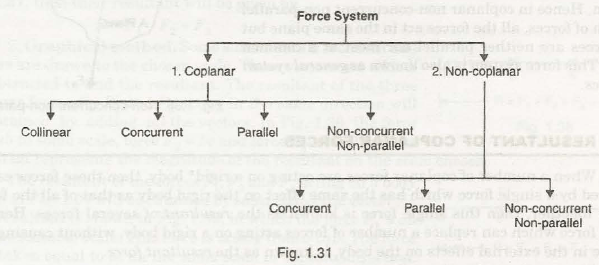We can see that coplanar and non-coplanar force systems further divide into several types of forces. In this chapter, we will discuss about coplanar forces and its types.

Coplanar system of force:

Coplanar forces are classified into four-

1. Coplanar Collinear Force:

When the line of action of all the forces is in the same straight line, same direction and in the same plane, it is known as coplanar collinear system of force as shown in Fig. 1.32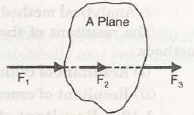Three forces F1, F2 and F3 are in a straight line on the same plane shows coplanar collinear force system.

1. Coplanar Concurrent Force

When two or more forces intersect at a single point on the same plane are called coplanar concurrent system of force as shown in Fig. 1.33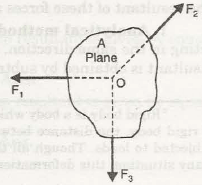Three forces F1, F2 and F3meetat a single point O on the same plane shows coplanar concurrent force system.

1. Coplanar Parallel Force

When two or more forces acting on a body are parallel to each other on the same plane, the force system is called coplanar parallel system of force as shown in Fig. 1.34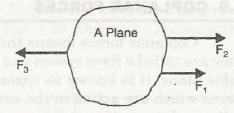Three forces F1, F2 and F3are parallel to each other but acts in different direction on the same plane shows coplanar parallel force system.

1. Coplanar Non-Concurrent Non-Parallel Force:

When several forces acting on a body are non-parallel and having different directions on the same plane, the force system is called coplanar non-concurrent non-parallel system of force as shown in Fig. 1.35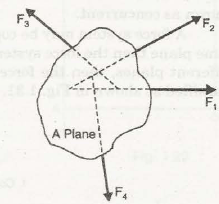In this force system, no force will meet at a single common point. Suppose four forces F1, F2, F3and F4 are non-parallel to each other and act in different direction on the same plane shows coplanar non-concurrent non-parallel force system.

Links of Next Mechanical Engineering Topics:-### Customer Reviews

My Homework Help
Rated 5.0 out of 5 based on 510 customer reviews at